# Methods for Reducing Ripple and Noise in Converter

For most systems, the variation of output voltage in power converter consists of two components, ripple and noise, which are both unavoidable considerations. Most of the variation can be minimized by external components, especially for high-precision specification.

This article describes the ripples voltage and noise voltage, and then introduces the common ways to suppress the ripples and noise of power converters, and how to achieve the effect of stabilizing the output voltage through the extra components.

1. Introduction

For the amplitude of output voltage in the flyback converter, the following will introduce that the components affect the ripple voltage and noise voltage, and the definition of the power supply rejection ratio at last.

1.1 Ripple Voltage

Ripple voltage is defined that the unexpected amount of AC voltage variation that occurs on a DC voltage power supply. The formula for the ripple voltage of the flyback converter is as shown in Equation (1). The following briefly introduces the parameters related to the ripple voltage.

It is known that the ripple voltage is inversely proportional to the output resistance(R), the output capacitor(C), and the switching frequency(f), but it is proportional to the duty cycle(D).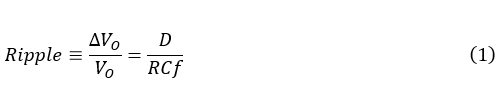The common specifications of flyback converter as the example, when the duty cycle is 50%, the output voltage is 12V, the load current is 1mA, the output capacitance is 1μF, and the switching frequency is 5kHz, which can be pushed out by (1).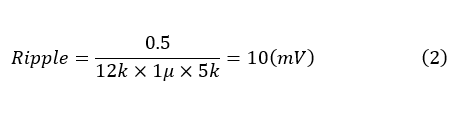• Switching Frequency

The size of electronic equipment is also gradually developing towards miniaturization, if the switching frequency is increased, not only the size of the overall system can be significantly reduced, but also ripple voltage become lower. But it will reduce the minimum magnetic inductance (Lm) during continuous operation, cause greater electromagnetic interference and lower conversion efficiency. such as equation (3)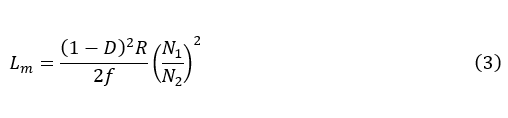where N1 and N2 are the number of coil-turns on the primary and secondary of the transformer, respectively.

• Output Capacitor

As the output capacitance and output resistance increase, the ripple voltage decreases. The approach will be needed larger size for specification. Therefore, it is obviously not suitable for the goal of miniaturization.

1.2 Noise Voltage

Whether interfered by the external environment or internal circuits, analog signals are easily affected from different frequency during transmission, which is called noise. Common noise voltage sources in flyback converter are discussed below.

• Ringing Phenomenon

In every circuit, each node will inevitably be parasitic inductance and parasitic capacitance. Hence, during the moment of power MOS switching, high-frequency ringing will occur, which is called switching noise as shown in figure 1:The ringing resonance frequency(fring)is susceptible to parasitic capacitance and parasitic inductance.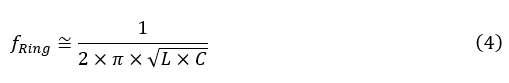A common solution is to select power transistors which have small parasitic capacitances, to reduce the parasitic capacitance and parasitic inductance of copper wires by optimizing the layout path of the printed circuit board specifically, and then using external components to absorb surge voltage.

• Power Supply Rejection Ratio

Power Supply Rejection Ratio (PSRR) is used to describe the power converter's ability to suppress power noise, and which is defined as the ratio of the change in the supply voltage to the change in output voltage, and

When the value is larger, which means that the better the suppression ability. The equation is following.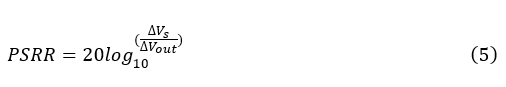It can be seen that the disturbance of power supply has a very serious impact on the output noise. In practice, the common countermeasures are that an external buffer or filter can be used to avoid problems such as low conversion efficiency.

2. How to Reduce Output Voltage Variation

There are quite common methods include using output capacitors, LC filters combined with capacitors and inductors, and low-dropout linear regulators. The following will description notices in the use of methods.

2.1 Capacitor

In figure 2, using directly an external capacitor which is parallel to power converter can suppress noise. The capacitor can be divided into three parts, namely static capacitance, equivalent series inductance (ESL), and equivalent series resistance (ESR). However, the static capacitance affects the stability of the output voltage; the value of ESL and ESR are proportional to voltage noise. In order to effectively reduce the output noise of the power converter, the key point is the impedance characteristics of the capacitor. Hence, not only selecting capacitors with good high-frequency characteristics meaning low ESL and ESR are such as multilayer ceramic capacitors, but also connecting multiple capacitors of the same capacity in parallel reduces equivalent series resistance (ESR), which can both achieve the purpose of suppressing voltage noise.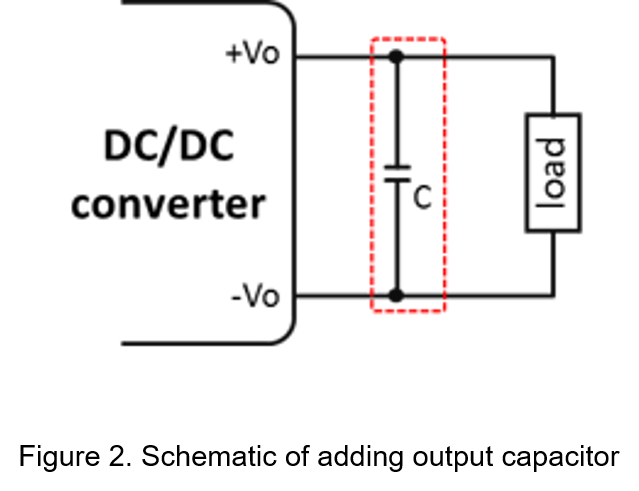2.2 LC Filter

In addition to providing the load current, the output capacitor can also eliminate ripple voltage. However, it cannot effectively suppress the low-frequency noise, so the inductor in series and the output capacitor in parallel which form an LC filter can solve problem.

The principle of LC filter is to allow signals which is in specific frequency band to pass completely, and then attenuate all signals outside this frequency band. In most actual cases, LC filter is to attenuate high-frequency interference signal strongly, and then only keep low-frequency signal to pass.

Generally, LC filter must be designed in the worst case. When the output loading current is instantaneously change from heavy-load to no-load, the energy originally stored in the inductor will be released, which inevitably impact the surrounding components and maybe destroy other circuit. Therefore, the withstand voltage of the power transistor and the output capacitor in power converter must be designed with the strictest conditions.

Another consideration is that the wire resistance of inductor can pull low the output DC voltage, and it will divide with load resistor, so choosing an inductor with a low parasitic resistance is importance.2.3 Low-Dropout Regulator

Adding a low dropout regulator in the output of power converter can greatly improve the power supply rejection ratio and reduce ripple and noise voltage, which can meet high stability specification of the circuit, such as clock generator and communication circuits, as shown in the figure 5.

The principle of the low dropout regulator is to compare the output voltage and the reference voltage by the internal feedback circuit, and then adjust the load current through power transistor to keep output voltage stable. The advantage of this method is that low output voltage and fast transient response; the disadvantage is that poor dissipate heat, so it is not suitable for heavy load applications.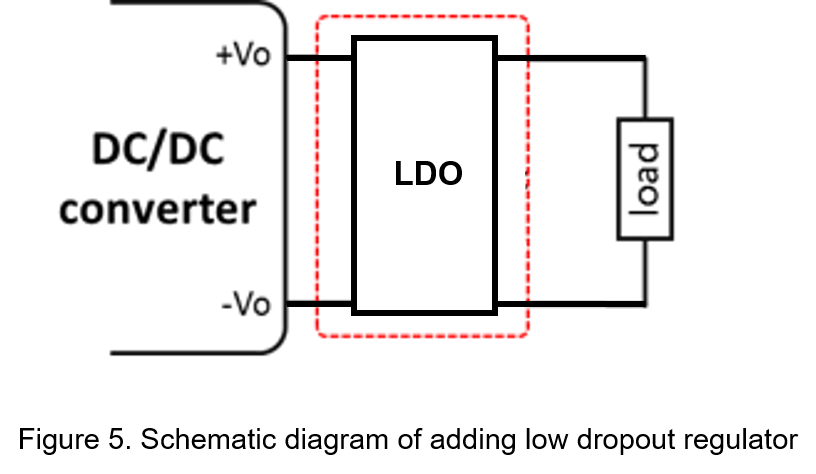3. Conclusion

Reducing ripple voltage and noise voltage is the direction of power converters with high accuracy and stability. The article analyzes the causes of ripple voltage and noise voltage, and provides three different circuit, namely capacitors, LC filters, and low dropout regulator to eliminate them.

In addition, the capacitor method just needs smallest size, but less helpful to reduce noise. On the contrary, the LC filter method also has small size and effectively suppresses ripple voltage, so it is widely used.

CTC is a professional service provider for high-end power supply modules (AC to DC Converter and DC to DC Converter) for critical applications worldwide since 30 years. Our core competence is to design and deliver products with leading technologies, competitive pricing, extremely flexible lead-time, global technical service and high-quality manufacturing (Made In Taiwan).

CTC is the only corporation certificated with ISO-9001, IATF-16949, ISO22613(IRIS), and ESD/ANSI-2020. We can 100% ensure not only the product, but also our workflow and service to match quality management system for every high-end application from the very beginning. From design to manufacturing and technical support, every single detail is operated under highest standard.